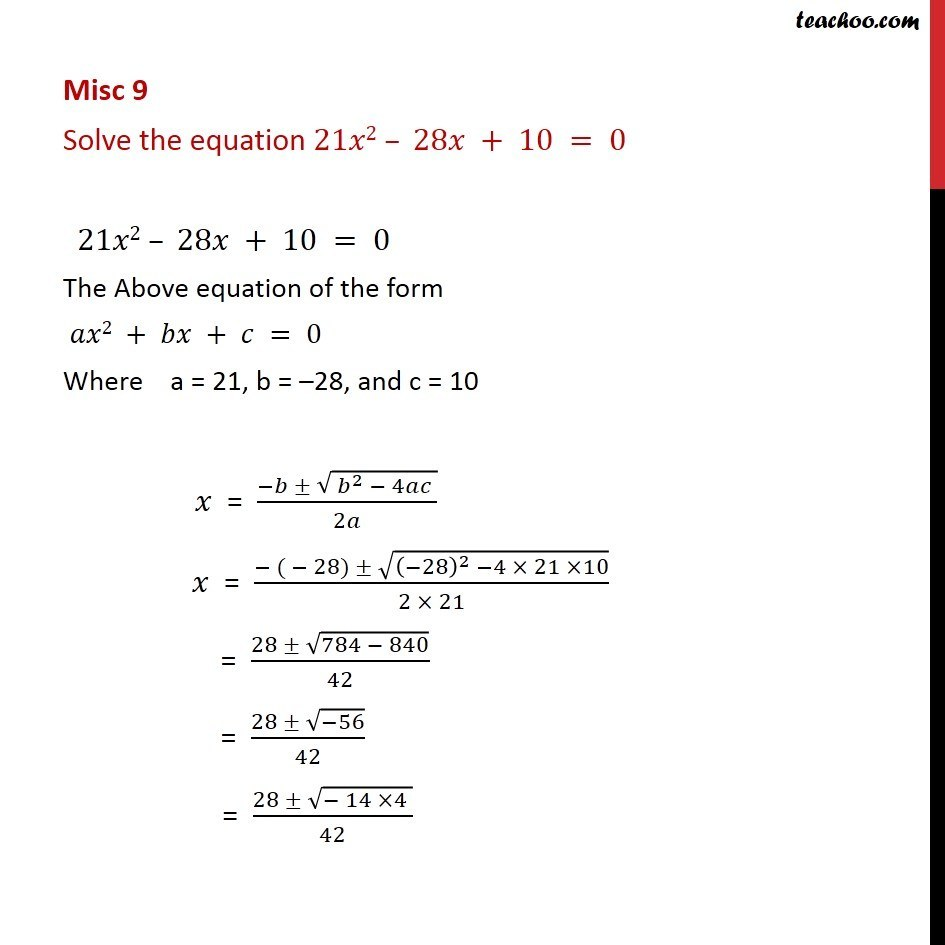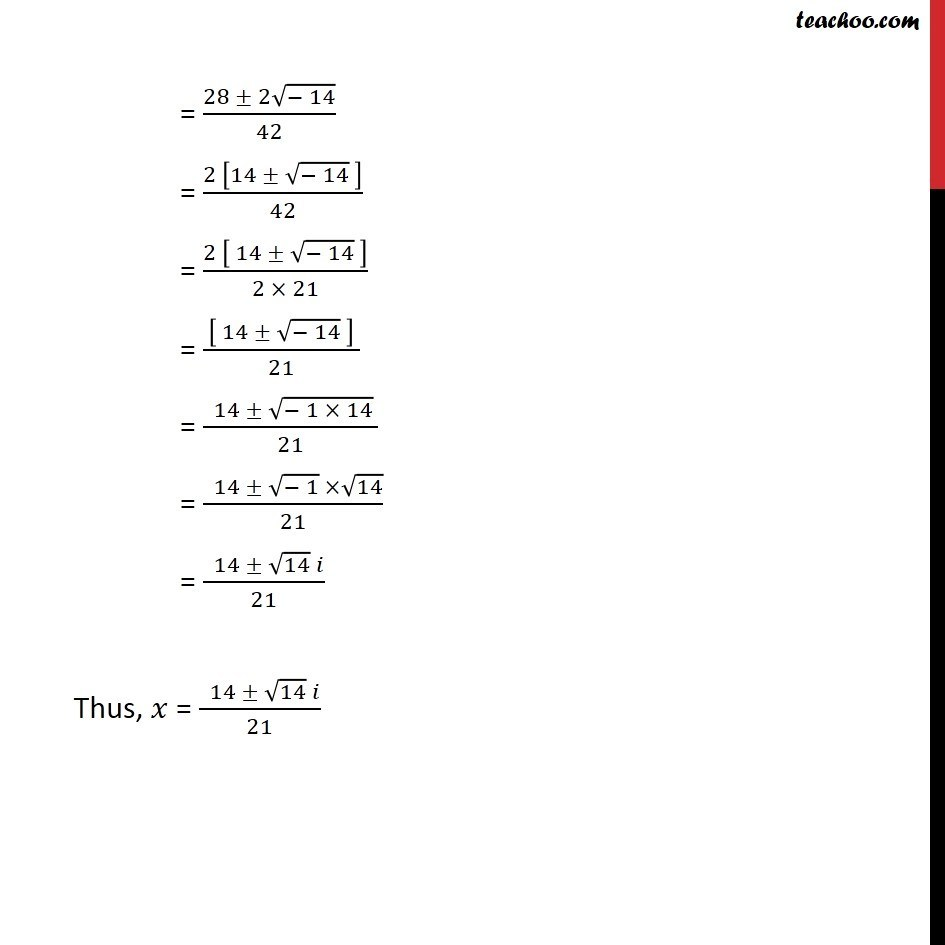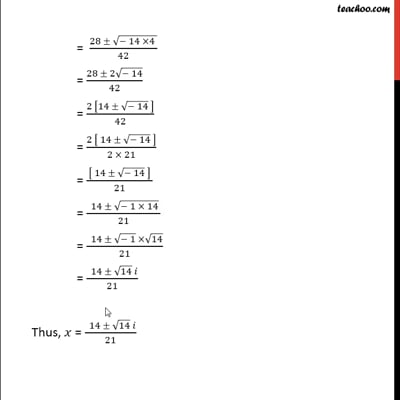Miscellaneous

Chapter 5 Class 11 Complex Numbers (Term 1)
Serial order wiseThis video is only available for Teachoo black users

### Transcript

Misc 9 Solve the equation 21𝑥2 – 28𝑥 + 10 = 0 21𝑥2 – 28𝑥 + 10 = 0 The Above equation of the form 𝑎𝑥2 + 𝑏𝑥 + 𝑐 = 0 Where a = 21, b = –28, and c = 10 𝑥 = (−𝑏 ± √( 𝑏^2 − 4𝑎𝑐 ))/2𝑎 𝑥 = (− ( − 28) ± √((−28)^2 −4 × 21 ×10))/(2 × 21) = (28 ± √(784 − 840))/42 = (28 ± √(−56))/42 = (28 ± √(− 14 ×4 ))/42 = (28 ± 2√(− 14))/42 = (2 [14 ± √(− 14) ])/42 = (2 [ 14 ± √(− 14) ])/(2 × 21) = ( [ 14 ± √(− 14) ] )/21 = ( 14 ± √(− 1 × 14) )/21 = ( 14 ± √(− 1) ×√14)/21 = ( 14 ± √14 𝑖)/21 Thus, 𝑥 = ( 14 ± √14 𝑖)/21